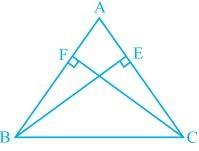Newbie

# BE and CF are two equal altitudes of a triangle ABC. Using RHS congruence rule, prove that the triangle ABC is isosceles. Q.4

• 0

Find this important question of ncert class 9th of chapter triangles .Sir please help me to find out the easiest and simplest solution of question 4 of exercise 7.3, give me the best solution of this question. BE and CF are two equal altitudes of a triangle ABC. Using RHS congruence rule, prove that the triangle ABC is isosceles.

Share

1..

Solution:

It is known that BE and CF are two equal altitudes.

Now, in ΔBEC and ΔCFB,

BEC = CFB = 90° (Same Altitudes)

BC = CB (Common side)

BE = CF (Common side)

So, ΔBEC ΔCFB by RHS congruence criterion.

Also, C = B (by CPCT)

Therefore, AB = AC as sides opposite to the equal angles is always equal.

• 0